# 22 in Binary

Language is a medium to communicate with each other or to express our feelings. Even computers have their own language, which is read in zeroes and ones. And the cool thing is that a word, a number, an image, or audio is converted into zeroes and ones.

In math, we follow a standard system to represent the numbers that are called a number system.

We have four different types of number systems with different bases. They are classified as: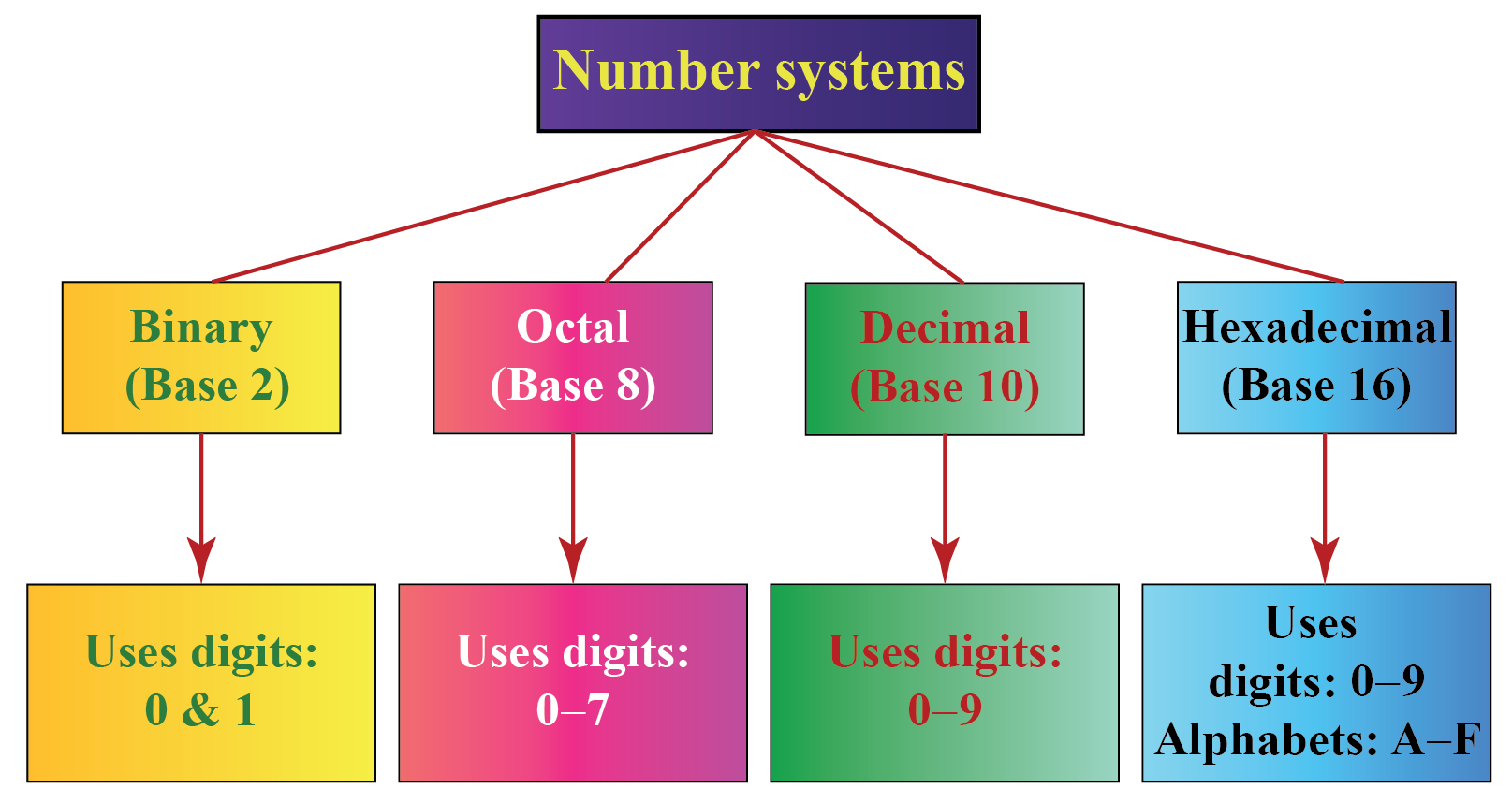In this mini-lesson, we will learn how to write 22 in its binary form.

## How Do You Convert 22 From Decimal to Binary?

Numbers are represented using the digits 0, 1, 2, ..., 9. This system where we use the digits 0, 1, 2, ..., 9 to write numbers is called the "decimal system."

Though we do not write any base to the numbers, the default base is 10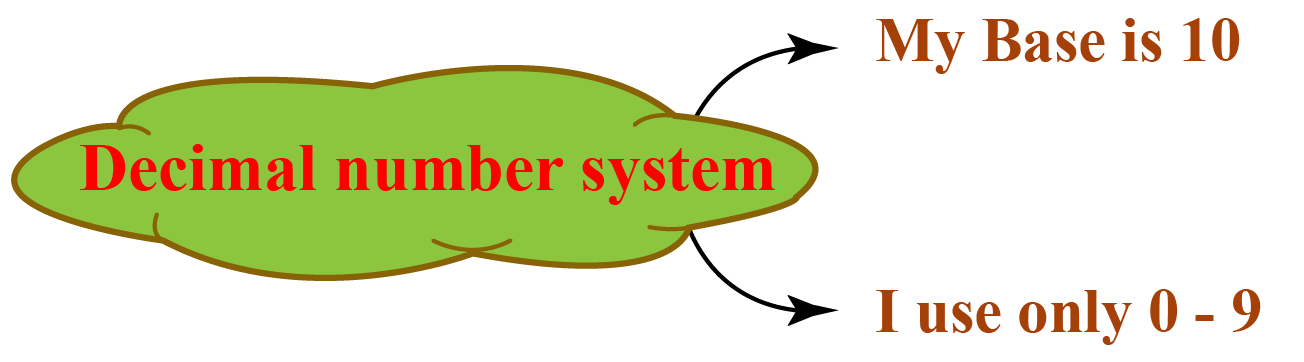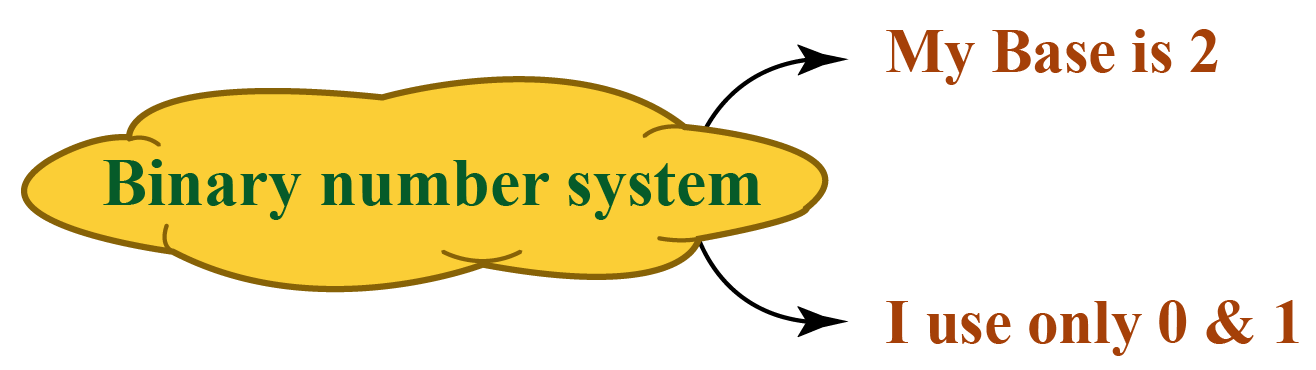Therefore, 22 can also be written as $$22_{10}$$ in a decimal representation.

Converting 22 from decimal to binary means to write or represent 22 using 2 bits only, i.e., 0 and 1 because the base-2 number system uses only 2 digits (0 and 1).

$$22_{10}$$ in binary is $$10110_{2}$$

## How Do I Convert 22 From Decimal to Binary?

Here, 22 has no base. So by default, its base is 10

The base of the binary system is 2

So we have to convert $$22_{10}$$ to a number with base 2 say $$X_{2}$$.

To convert 22 into binary, we have to divide 22 by 2 and note down the quotient and the remainder in the “quotient-remainder” form.

Repeat this process (dividing the quotient again by 2) until we get the quotient to be less than the base.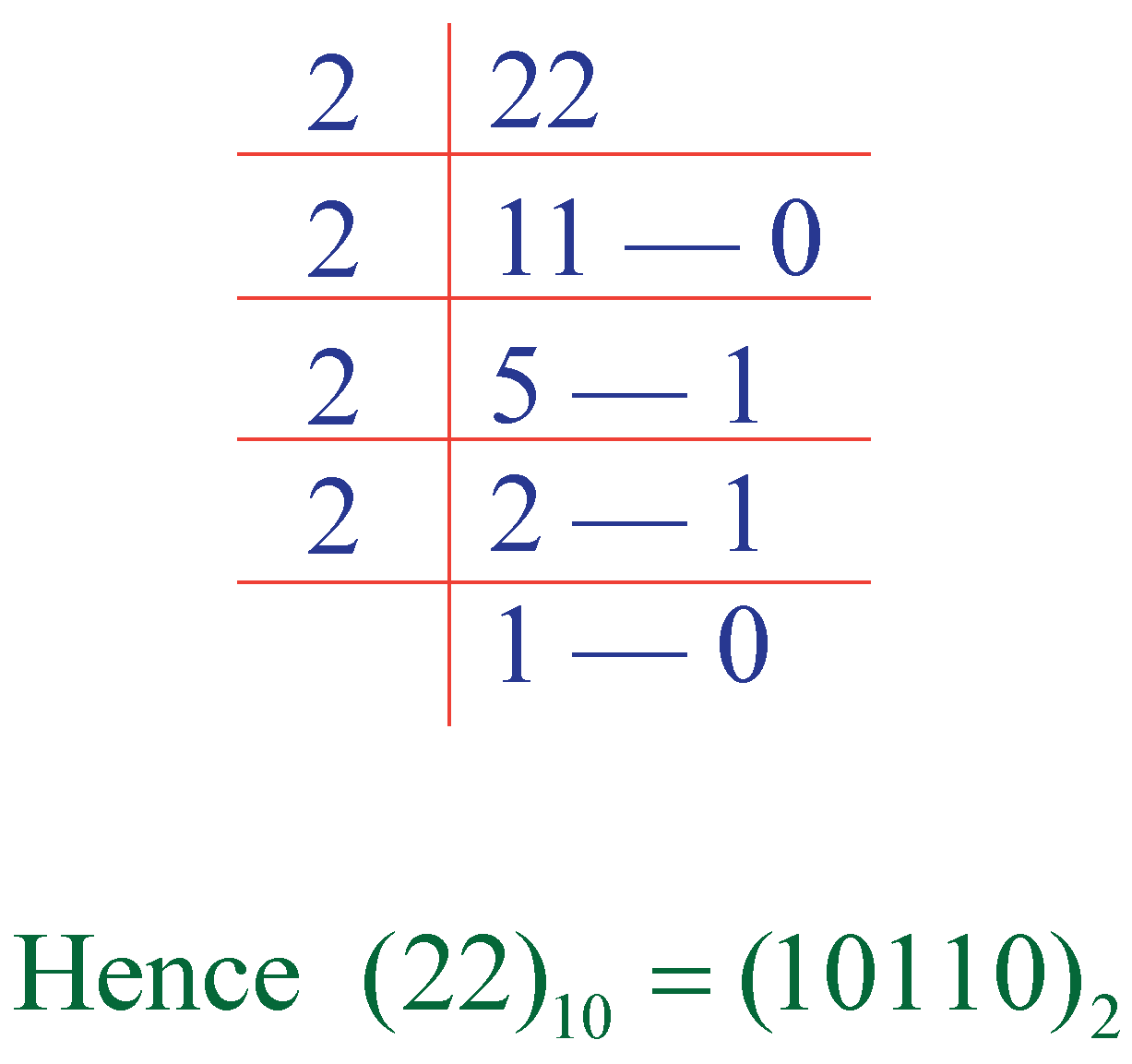22 in the binary system is obtained just by noting down all the remainders and the last quotient from bottom to top.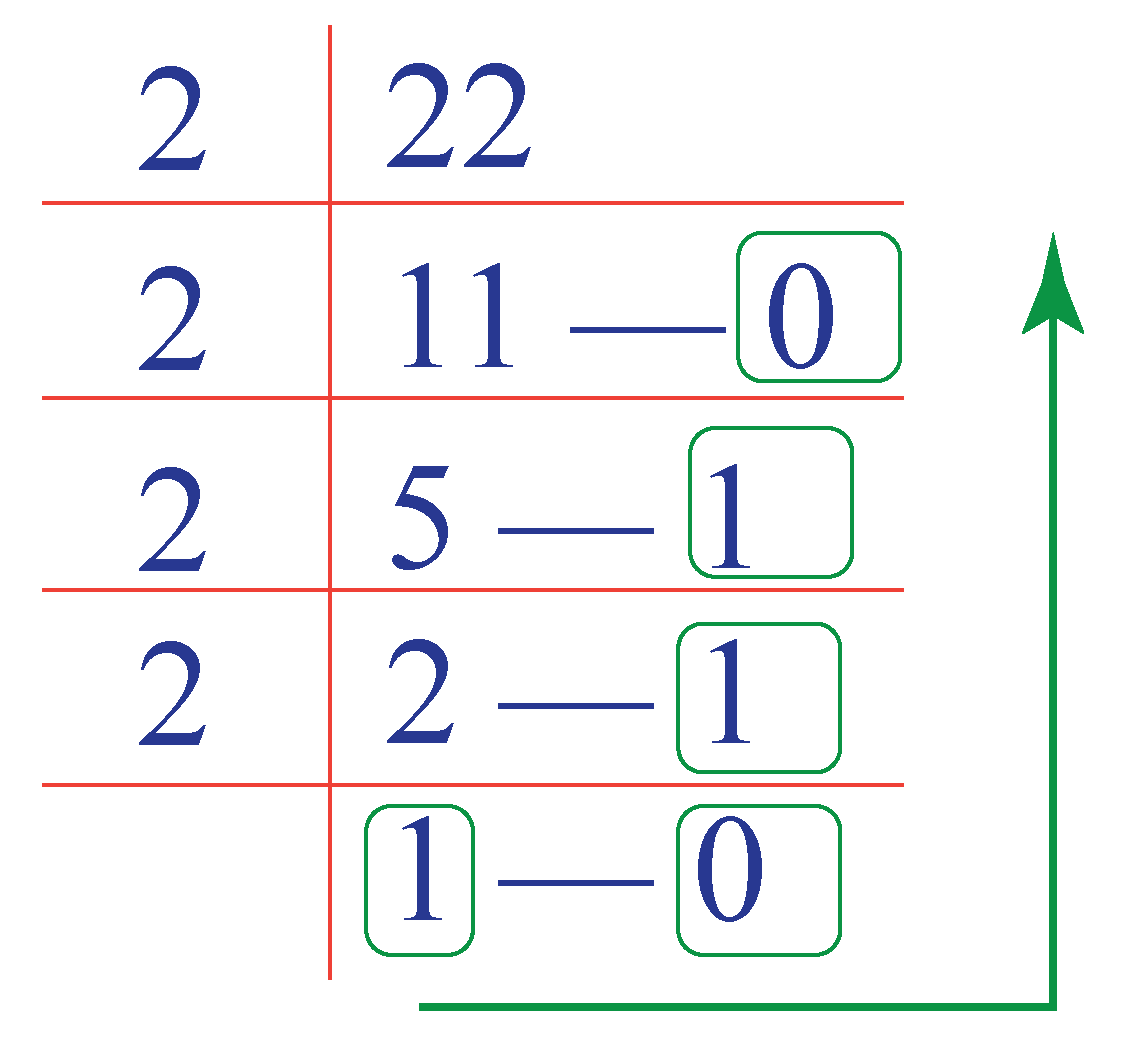Thus, 22 in binary is:

 22 (or) $$22_{10}$$ = $$10110_{2}$$Important Notes
• Sometimes the base is also referred to as 'Radix"
• Base-2 number system uses only 2 digits (0 and 1), base-8 number system uses 8 digits (0-7), base-10 number system uses digits from (0-9), and base-10 number system uses digits from (0-9) and alphabets (A-F).

## Solved Examples

 Example 1

Isabella enters the number 19 on a computer. How will the computer convert it into binary and store it?

Solution

To convert 19 into binary, the computer follows the given steps:

Step 1: Divide 19 by 2 to find the quotient and remainder.

Step 2: Divide the quotient obtained again by 2

Step 3: Repeat the process until the quotient becomes lesser than 2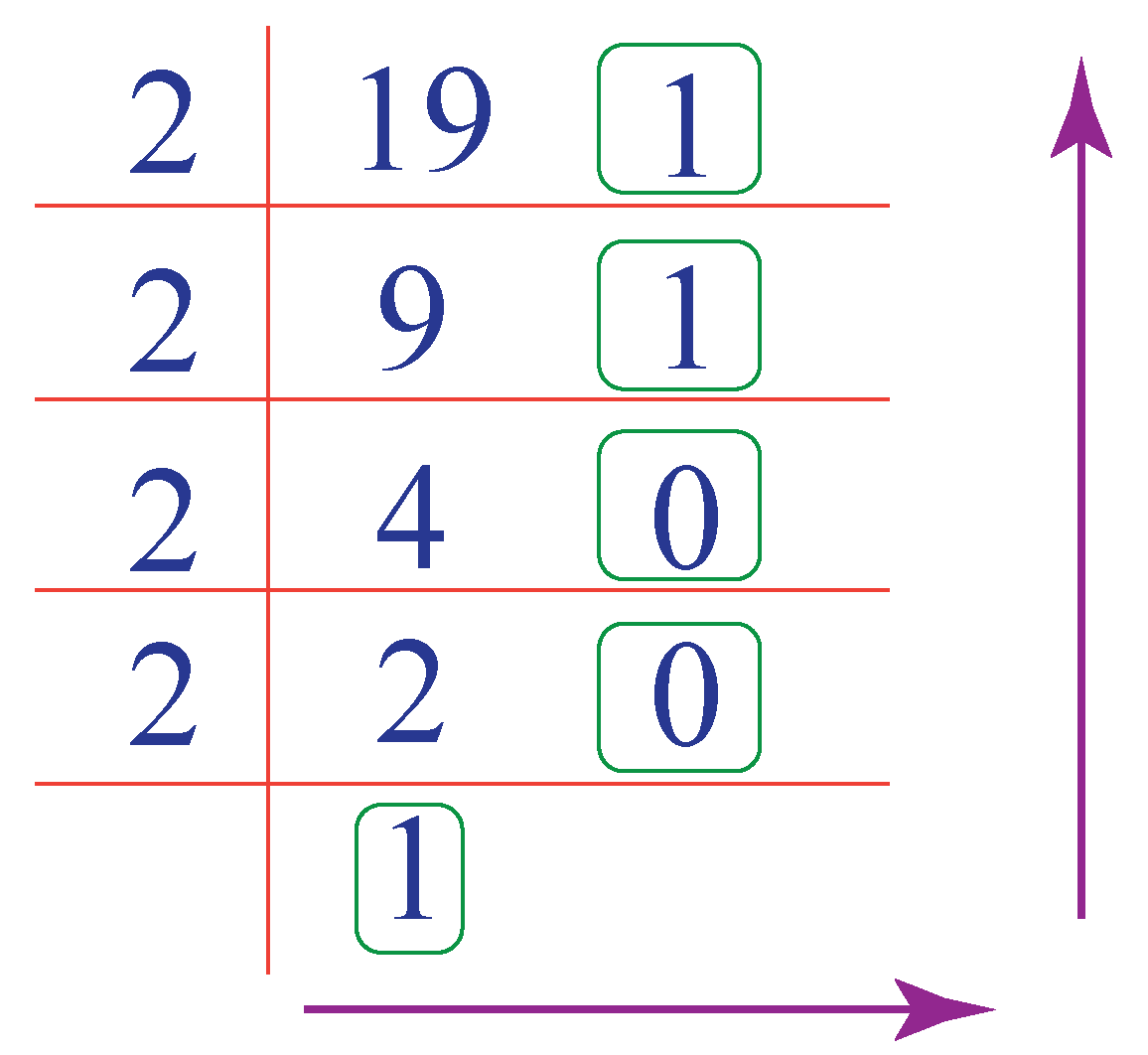$$\therefore$$ 19 in binary  = $$10011_2$$
 Example 2

William wants to write his age in binary. He is 15 years old. Can you help him write his age in binary?

Solution

15 can be converted into binary by the given steps:

Step 1: Divide 15 by 2 to find the quotient and remainder.

Step 2: Divide the quotient obtained again by 2

Step 3: Repeat the process until the quotient becomes lesser than 2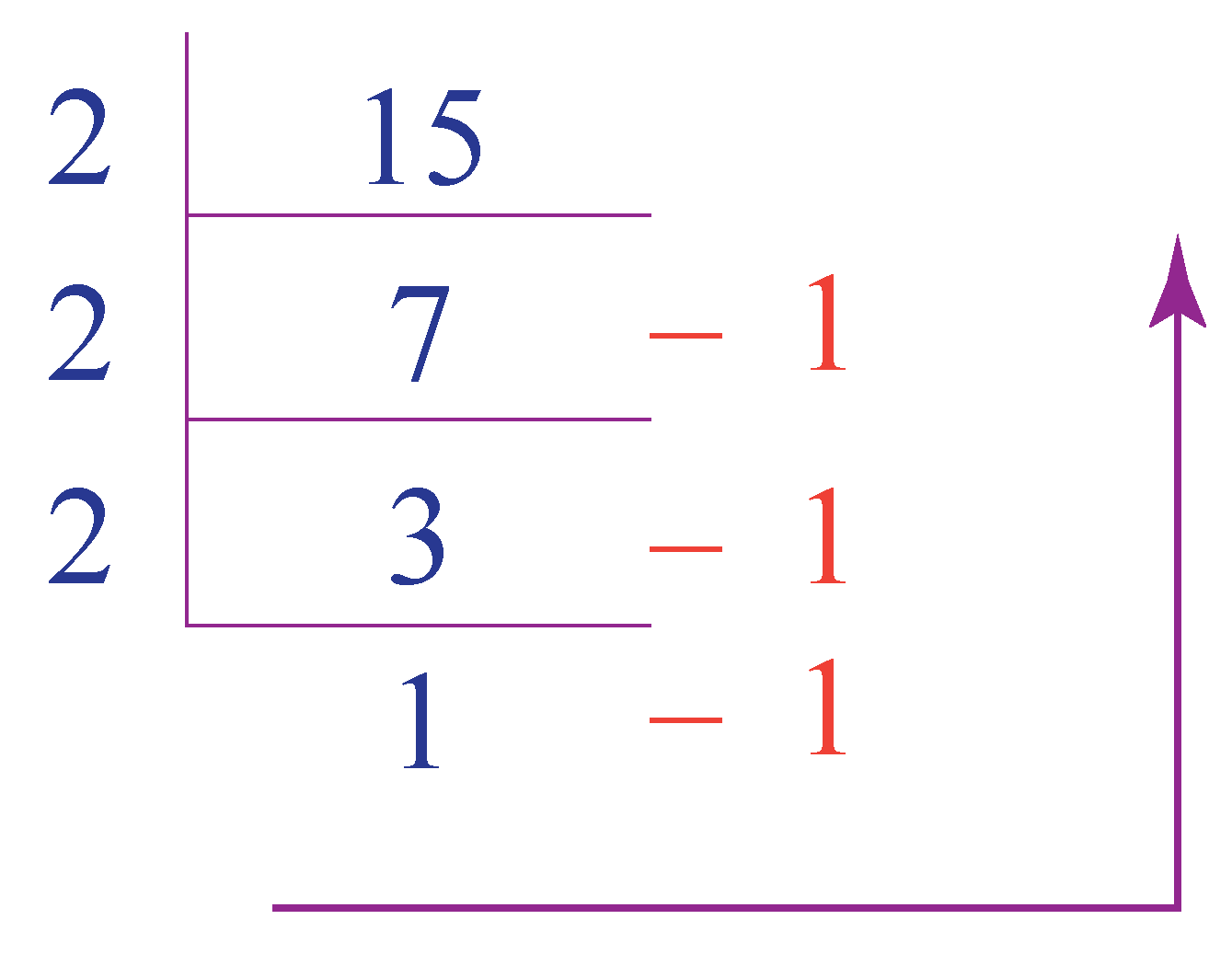$$\therefore \text { Age of William in binary } =1111 _2$$Challenging Questions

In this lesson, we have learned how to convert 22 in binary. Can you now try to convert the following binary numbers into corresponding numbers with decimal bases?

1. $$10100_2$$
2. $$10000_2$$
3. $$11011_2$$

## Interactive Questions

Here are a few activities for you to practice.

## Let's Summarize

The mini-lesson targeted the fascinating concept of writing 22 in binary. The math journey around the number 22 in binary starts with what a student already knows, and goes on to creatively crafting a fresh concept in the young minds. Done in a way that not only it is relatable and easy to grasp, but also will stay with them forever. Here lies the magic with Cuemath.

At Cuemath, our team of math experts is dedicated to making learning fun for our favorite readers, the students!

Through an interactive and engaging learning-teaching-learning approach, the teachers explore all angles of a topic.

Be it worksheets, online classes, doubt sessions, or any other form of relation, it’s the logical thinking and smart learning approach that we, at Cuemath, believe in.

## 1. How do you write 2 in binary?

2 in binary is written as 10

## 2. What is the binary number for 22?

22 in binary is written as 10110

## 3. What number is 10101 in binary?

Reading binary code means converting the binary number into the decimal number system so that it can be easily understood as we are more familiar with this number system.

Hence, $$10101_2$$ will be read as 21 since it is the decimal system equivalent of $$10101_2$$

More Important Topics
Numbers
Algebra
Geometry
Measurement
Money
Data
Trigonometry
Calculus